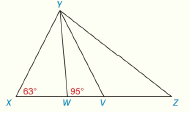Chapter 2.CT, Problem 19CT### Elementary Geometry for College St...

6th Edition
Daniel C. Alexander + 1 other
ISBN: 9781285195698

#### Solutions

Chapter
Section### Elementary Geometry for College St...

6th Edition
Daniel C. Alexander + 1 other
ISBN: 9781285195698
Textbook Problem
1 views

# In Δ X Y Z , ∠ X Y Z is trisected by Y W ¯ and Y V ¯ . With angle measures as shown, find m ∠ Z .____________To determine

To find:

The value of mZ.

Explanation

Given:

The following figure shows the given diagram.

Given that, in ΔXYZ, XYZ is trisected by YW¯ and YV¯.

Concept:

The sum of a linear pair is 180°.

In a triangle, sum of all interior angles is 180°.

Calculation:

XWY and YWV are forming linear pair.

Write the sum of angle as shown below.

XWY+YWV=180°

Substitute 95° YWV in the above obtained equation.

XWY+95°=180°XWY=180°95°=85°

Consider the ΔXWY.

WXY+XYW+XWY=180°.

Substitute 63° for XYZ, and 85° for XWY in the above obtained equation

### Still sussing out bartleby?

Check out a sample textbook solution.

See a sample solution

#### The Solution to Your Study Problems

Bartleby provides explanations to thousands of textbook problems written by our experts, many with advanced degrees!

Get Started

#### Find more solutions based on key concepts